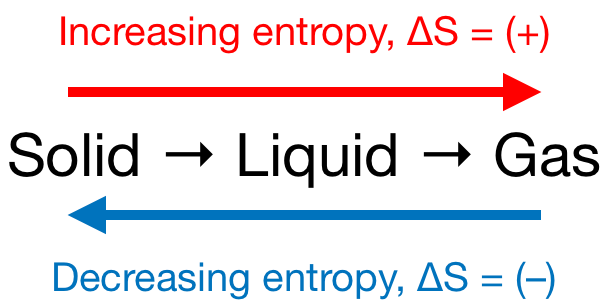# Problem: Without doing any calculations, determine the sign of ΔSsys for each of the following chemical reactions.a. Mg(s) + Cl2(g) → MgCl2(s)b. 2 H2S(g) + 3 O2(g) → 2 H2O(g) + 2 SO2(g)c. 2 O3(g) → 3 O2(g)d. HCl(g) + NH3(g) → NH4Cl(s)

###### FREE Expert Solution

We’re being asked to predict the sign of the entropy change (ΔS) for the given reactions

Recall that if:

• ΔS > 0 or ΔS = (+); entropy is increased

• ΔS < 0 or ΔS = (–); entropy is decreased

Remember that phase changes lead to a change in entropy:Also, recall that an increase in the number of gas molecules in the reaction also leads to an increase in entropy.

97% (154 ratings)###### Problem Details

Without doing any calculations, determine the sign of ΔSsys for each of the following chemical reactions.
a. Mg(s) + Cl2(g) → MgCl2(s)
b. 2 H2S(g) + 3 O2(g) → 2 H2O(g) + 2 SO2(g)
c. 2 O3(g) → 3 O2(g)
d. HCl(g) + NH3(g) → NH4Cl(s)